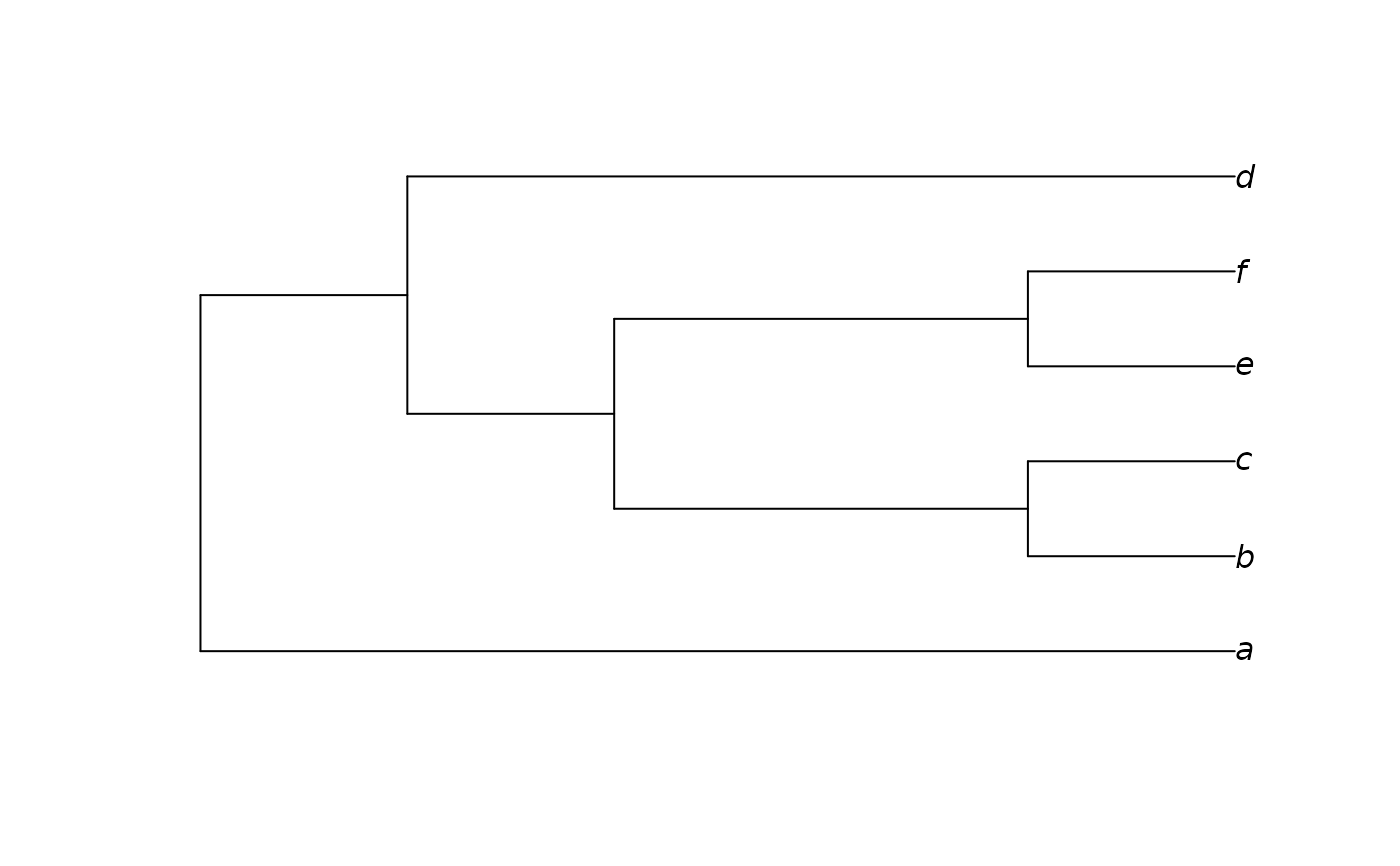Constructs an approximation to a neighbour-joining tree, modified in order to be consistent with a constraint. Zero-length branches are collapsed at random.

ConstrainedNJ(dataset, constraint, weight = 1L, ratio = TRUE, ambig = "mean")

## Arguments

dataset

A phylogenetic data matrix of phangorn class phyDat, whose names correspond to the labels of any accompanying tree.

constraint

Either an object of class phyDat, in which case returned trees will be perfectly compatible with each character in constraint; or a tree of class phylo, in which each node in constraint will occur in the returned tree. See vignette for further examples.

weight

Numeric specifying degree to up-weight characters in constraint.

ambig, ratio

Settings of ambig and ratio to be used when computing Hamming() distances between sequences.

## Value

ConstrainedNJ() returns a tree of class phylo.

Other tree generation functions: GenerateTree, NJTree(), TreeNumber, TrivialTree

## Author

Martin R. Smith (martin.smith@durham.ac.uk)

## Examples

dataset <- MatrixToPhyDat(matrix(
c(0, 1, 1, 1, 0, 1,
0, 1, 1, 0, 0, 1), ncol = 2,
dimnames = list(letters[1:6], NULL)))
constraint <- MatrixToPhyDat(
c(a = 0, b = 0, c = 0, d = 0, e = 1, f = 1))
plot(ConstrainedNJ(dataset, constraint))